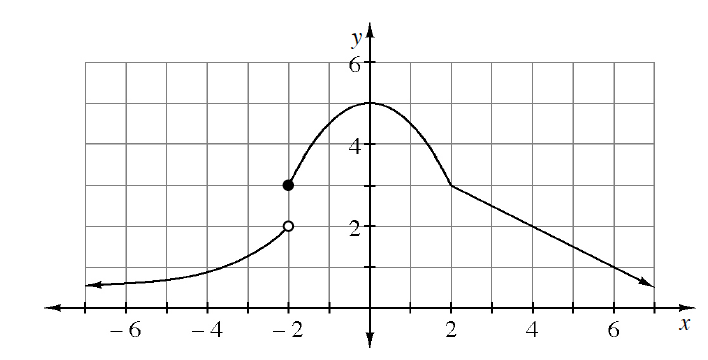### Home > PC3 > Chapter 7 > Lesson 7.1.3 > Problem7-36

7-36.

Given the graph of the piecewise-defined function at right, complete the following limit statements or state that the limit does not exist.1. $\lim\limits _ { x \rightarrow - \infty } f ( x ) =$

What happens to the curve on the far left side?

2. $\lim\limits _ { x \rightarrow - 2 ^ { - } } f ( x ) =$

What is the height of the function if you approach $x=-2$ from the left?

3. $\lim\limits _ { x \rightarrow - 2 ^ { + } } f ( x ) =$

What is the height of the function if you approach $x=-2$ from the right?

4. $\lim\limits _ { x \rightarrow - 2 } f ( x ) =$

5. $\lim\limits_ { x \rightarrow 2 } f ( x ) =$
Since the curve is continuous at $x=2$, this is just $f(2)$.
6. $\lim\limits _ { x \rightarrow \infty } f ( x ) =$